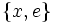# Derived subgroup of dihedral group:D16

Jump to: navigation, search
This article is about a particular subgroup in a group, up to equivalence of subgroups (i.e., an isomorphism of groups that induces the corresponding isomorphism of subgroups). The subgroup is (up to isomorphism) cyclic group:Z4 and the group is (up to isomorphism) dihedral group:D16 (see subgroup structure of dihedral group:D16).
The subgroup is a normal subgroup and the quotient group is isomorphic to Klein four-group.
VIEW: Group-subgroup pairs with the same subgroup part | Group-subgroup pairs with the same group part| Group-subgroup pairs with the same quotient part | All pages on particular subgroups in groups

## Definition

Here,$G$ is the dihedral group:D16, the dihedral group of order sixteen (and hence, degree eight). We use here the presentation:$G := \langle a,x \mid a^8 = x^2 = e, xax = a^{-1} \rangle$$G$ has 16 elements:$\! \{ e,a,a^2,a^3,a^4,a^5,a^6,a^7,x,ax,a^2x,a^3x,a^4x,a^5x,a^6x,a^7x \}$

The subgroup$H$ of interest is the subgroup$\langle a^2 \rangle$. It is cyclic of order 4 and is given by:$H := \{ e, a^2, a^4, a^6 \}$

The quotient group is a Klein four-group.

## Cosets

The subgroup is a normal subgroup, so its left cosets coincide with its right cosets. There are four cosets:$\! \{ e,a^2,a^4,a^6\}, \{ a, a^3, a^5, a^7 \}, \{ x, a^2x, a^4x, a^6x \}, \{ ax, a^3x, a^5x, a^7x \}$

## Arithmetic functions

Function Value Explanation
order of whole group 16
order of subgroup 4
index of subgroup 4
size of conjugacy class (=index of normalizer) 1
number of conjugacy classes in automorphism class 1

## Subgroup-defining functions

Subgroup-defining function Meaning in general Why it takes this value
derived subgroup subgroup generated by all commutators The commutators are precisely the elements of this subgroup. For instance,$xax^{-1}a^{-1} = a^{-2}$.
first agemo subgroup subgroup generated by all$p^{th}$ powers. Here,$p = 2$, so subgroup generated by squares
Frattini subgroup intersection of all maximal subgroups
Jacobson radical intersection of all maximal normal subgroups

## Subgroup properties

### Invariance under automorphisms and endomorphisms

Property Meaning Satisfied? Explanation
normal subgroup invariant under inner automorphisms Yes derived subgroup is normal
characteristic subgroup invariant under all automorphisms Yes derived subgroup is characteristic
fully invariant subgroup invariant under all endomorphisms Yes derived subgroup is fully invariant, agemo subgroups are fully invariant
isomorph-free subgroup no other isomorphic subgroup Yes
verbal subgroup generated by set of words Yes derived subgroup is verbal, agemo subgroups are verbal
homomorph-containing subgroup contains every homomorphic image No The subgroup$\{ x, e \}$ is an image of this subgroup but is not contained in it.

## GAP implementation

The group and subgroup can be constructed using GAP's SmallGroup and DerivedSubgroup functions:

G := DihedralGroup(16); H := DerivedSubgroup(G);

The GAP display looks as follows:

gap> G := DihedralGroup(16); H := DerivedSubgroup(G);
<pc group of size 16 with 4 generators>
Group([ f3, f4 ])
Here is a GAP implementation to verify some of the assertions made in this page:[SHOW MORE]